# Evaluation of the Control Strategy Performance for Isolated Variable-Speed Wind Turbine Using Different Wind Speed Models at Different Load Cases under Balanced/Unbalanced Excitation

Evaluation of the Control Strategy Performance for Isolated Variable-Speed Wind Turbine Using Different Wind Speed Models at Different Load Cases under Balanced/Unbalanced Excitation

Saber M. SalehAsmaa S. Farag

Electrical Engineering Dept. Faculty of Engineering, Fayoum University, Fayoum, Egypt

Corresponding Author Email:
sabermssh@gmail.com
Page:
341-353
|
DOI:
https://doi.org/10.18280/ejee.210401
13 April 2019
|
Accepted:
29 July 2019
|
Published:
3 October 2019
| Citation

OPEN ACCESS

Abstract:

The evaluation of the proposed control strategy performance is needed to ensure that, it is applicable. This paper presents a complete control strategy for region two and region three of a stand-alone SEIG driven by a VSWT. This strategy evaluated using realistic and random wind model at different load cases under balanced/unbalanced excitation. In region two for speed range from cut-in to rated speed, the MPPT using torque control strategy is used, while in region three for speed from rated speed to cut-out, pitch control using gain scheduling PI controller is used. Comparison between conventional and gain scheduling PI is presented. For pitch control to clarify the pitch angle required for each speed, a step function is used. The realistic wind speed, the wind turbine, and the SEIG models have been simulated by using the MATLAB/SIMULINK program based on the dynamic equations of each model. The influence of the speed variation on the steady state generated voltage and the rotor speed are studied. A dynamic study indicates that the proposed strategy is stable, fulfillment, and reliable for real time applications.

Keywords:

variable speed wind turbine (VSWT), self-excited induction generator (SEIG), maximum power point tracking (MPPT), torque control, pitch angle control, realistic wind model, random wind model, balanced/unbalanced excitation

1. Introduction

Maximum extracted power from the wind needs VSWTs needs variable pitch blades to capture more wind power and achieve higher power qualities. During the past few years, the VSWT has become the dominant type among the installed WTs Compared with fixed speed wind turbine (FSWT), VSWTs offer many benefits as opposed to FS ones although the FS system is easy to be built and operate. The major advantage of VSWTs is increasing the energy that can be captured since their annual energy production can reach up to 20 % increase over FSWTs [1, 2]. In addition, they have many advantages such as reduction in transient mechanical load and better power conditioning due to intelligent control strategies can be used for them. It has also been recognized that advanced controllers in such WTs play the important role in the performance and the attenuation of the unfavorable effects of loads on the large WTs structure. Moreover, the VS system is much more complex to control. This allows better use of resources of the WT, increasing the lifetime of mechanical and electrical components, earning higher returns. These types are better grid compliant compared to the FSWTs. There is a possibility to independently control the speed decoupled from the grid, gives the possibility to decouple frequencies resulting from the wind fluctuations from the grid, which reduces flicker contribution. In addition, a full-scale power converter integrated between the generator and the grid in VSWTs gives the possibility to actively control the power output of the WT, which is increasingly important for the integration of WTs into the grid. It also enables an efficient power production at a huge range of different wind speeds since the mechanical speed is decoupled from the unified network frequencies. This may give a speed variation of up to 120 %. The success of this concept has been limited over many years due to technical development in the area of power electronics and associated costs . In recent years, VSWTs with induction or synchronous generator becomes very important connected either directly or indirectly to the grid. The control strategies for any kind of WT play a major role in its characteristics. The operating regions in VSWT are classified into two major categories which are below and above rated speed of the wind [4, 5].

There are many previous attempts to design and control performance evaluation for research or applied purpose. The following table compares between the previous study and the proposed control performance evaluation.

In this work, multivariable control strategies for the VSWT and variable pitch WTs have been investigated. The main target of the controller is to optimize the wind energy capture (maximum power point tracking MPPT) below rated wind speed by controlling the generator torque is included in section 4. However, above-rated wind speed controller (i.e. pitch control) target is to maintain the rated power of the WT. So, robust control of a VS pitch WT is introduced in section 5. Simulation results for the proposed control strategies have been investigated in section 6. The proposed Wind energy conversion system (WECS) is also studied under different load cases and for balanced and unbalanced excitation cases. Section 7 concludes the paper.

2. Model of the System under Studying

The MATLAB/SIMULINK program, as shown in Figure 1, used to simulate the realistic wind speed, the wind turbine, and the self-excited induction generator (SEIG) models based on the dynamic equations of each model [16, 17].

Table 1. Comparison between the proposed method and the previous study

 Variable -Speed Region 2 Region 3 Power system model realistic wind model Random wind model Different load cases balanced/ unbalanced excitation Proposed Ok Ok Ok Ok Ok Ok Ok Ok Ref.  No No Ok No No No No No Ref.  Ok Ok No No Ok No No No Ref.  Ok Ok No Ok No No No No Ref.  Ok Ok No Ok No No No No Ref.  No No Ok No No Ok No No Ref.  Ok No Ok No No No No No Ref.  Ok Ok No Ok No No No No Ref.  Ok Ok No Ok No No Ok No Ref.  Ok Ok Ok No Ok No No No Ref.  Ok Ok No Ok Ok No Ok No

## 1.png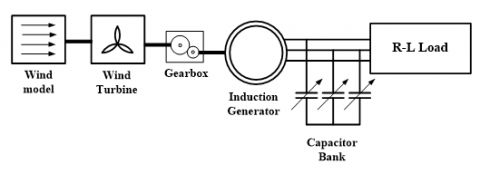Figure 1. Schematic diagram for the system under study

3. Variable-Speed Wind Turbines

To be more adaptable to the variation in the wind speed and to be more efficient, most modern utility-scale WTs operate in VS mode with the WT speed changing continuously in response to wind gusts and lulls. This is because as the wind speed varies, the mechanical power on the generator shaft cannot be kept constant. Furthermore, effects like tower shadow, an uneven distribution of wind speed over the rotor disc and mechanical oscillations in the drive train contribute to variations in mechanical power . The VSWTs are operated with VS generators, so it must incorporate a generator control system that can operate with VS. To fulfill such a requirement, these generators must be connected to the grid via power electronic converters. However, FSWTs are connected directly to the utility grid, which eliminates the requirement for power electronics.  Although costly power electronics are required to convert the variable-frequency power to the fixed unified network grid frequency, the VSWTs can operate at maximum aerodynamic efficiency more time than FSWTs. In addition, VSWTs often endure smaller power fluctuations and operating loads than FSWTs. For modern WTs and power electronics systems, the increased efficiency and lower loads of VSWTs provide enough benefit to make cost-effective of the power electronics. They are designed to achieve maximum aerodynamic efficiency over a wind speeds wide range. Keeping the tip speed ratio (λ) constant in Eq. (1) is required for maximum power coefficient (Cpm). Contrary to the FS system, the generator torque kept fairly constant in a VS system while controlled the power converted. Then, the power fluctuations caused by variations of wind speed are absorbed by generator speed changes and consequently in the WT rotor speed. The result is fairly constant electric power without any significant variations. VSWT electrical systems are complex compared with the FSWT. The VSWT used with synchronous/induction generator connected to the network using a power converter .

$\mathrm{TSR}=\lambda=\frac{\mathrm{v}_{\mathrm{t}}}{\mathrm{v}_{\mathrm{w}}}=\frac{\omega_{\mathrm{t}} \mathrm{R}}{\mathrm{v}_{\mathrm{w}}}$ (11)

From all the above discussions, the VSWTs have advantages of increased energy captured; the mechanical stress reduced on the WT and improved power quality since it reduces the power fluctuations sent to the utility. However, their disadvantages are losses and cost of power electronics components. The WT output power varies with the cubic of wind speed as indicated in Eq. (2). Since the wind can change speed more quickly than the turbine, there is not a static relationship between wind speed and WT power in dynamic conditions. However, under steady-state conditions, a static relationship exists. Every WT has a characteristic power performance curve. A power curve example for hypothetical VSWT is presented in Figure 2 , showing the power curve available in the wind for this WT.

$\mathrm{P}_{\mathrm{t}}=0.5 \rho \mathrm{AC}_{\mathrm{p}}(\lambda, \beta) \mathrm{v}_{\mathrm{w}}^{3}=0.5 \rho \mathrm{AC}_{\mathrm{p}}(\lambda, \beta)\left(\frac{\omega_{t} \mathrm{R}}{\lambda}\right)^{3}$ (2)

## 2.pngFigure 2. Wind power, turbine power and operating regions for hypothetical WT

In Figure 2, the blue wind power curve represents the power in the unimpeded wind passed through the blades swept area which calculated from Eq. (3), whereas the green curve represents the extracted power by a hypothetical VSWT which calculated from Eq. (4). In this example, the WT produces maximum power (5000 kW) at 12 m/s rated wind speed, and it has maximum power coefficient Cpmax = 0.48. From the figure, the VSWT attempts to maximize captured energy while operating in region 2. However, in region 3 the power is limited to its rated power to ensure the loads are not exceeded. For each region, the green curve in the figure illustrates the desired power against wind speed relationship. The WT generator operation can be divided into four primary regions on the velocity scale that are labeled on the WT power curve .

$\mathrm{P}_{\mathrm{w}}=\frac{1}{2} \rho \mathrm{Av}^{3}$ (3)

$\mathrm{P}_{\mathrm{t}}=\frac{1}{2} \rho \mathrm{A}_{\mathrm{t}} \mathrm{v}_{1}^{3} \mathrm{C}_{\mathrm{p}}$ (4)

VSWTs have two main regions of operation that required control which is region 2 and region 3 . Therefore, about each region characteristic, the operation control levels can be classified as:

1) Extract maximum power during region 2.

2) Reduced the power to the rated value during region 3.

3) Mitigate the fatigue loads on the WT mechanical components.

WTs usually have at least three different possible control actuators which are generator torque, blade pitch, and machine yaw. In region 2 the generator torque control is usually used to maintain the WT operates at maximum Cpmax and add damping to the drivetrain torsion modes of the WT in region 3. While blades pitch control is used to limit power and speed on both the low-speed shaft and high-speed shaft for WTs operating in region 3 and the WT power output can be limited by yawing the machine out of the wind that way decreasing the projected rotor area and reducing power. Most often, yaw control is used only to respond at changes of the wind direction in an attempt to reduce the yaw error (the angle between the mean wind direction and the orientation direction of the WT) and thereby maximize power .

4. Wind Speed Below Rated (Region 2) Control Strategy

In this region, the WS is below its rated value and the electrical output power is below its rated value as in Figure 2. Then, the main objective of the controller in this region is to maximize the wind energy captured. To maintain Cp at its maximum value, and hence maximize the wind energy captured, we use MPPT control strategy. The goal of the (MPPT) strategy is extracted maximum power from the wind.

The captured power by the WT may be written as in Eq. 4. From this equation, the power production can be maximized from the WT at maximum CP. The WT can't capture the full available power from the wind but it can only extract apart, which means it can't operate at CP =1. 100 % efficiency cannot be achieved because some kinetic energy must remain after the wind passed through WT, otherwise, the wind will be stopped with no more wind will pass through the WT rotor to provide energy. In fact, the Betz limit is the theoretical maximum aerodynamic efficiency is approximately 59 % of the wind power. In addition, because aerodynamic losses the target is to approach the Betz Limit curve as possible. The proposed WT for this paperwork can achieve maximum Cpmax =0.48.

A Cp is non-linear and also varies with λ, which is a function of wind speed and WT rotational speed, and the blade parameters of the WT such as pitch angle β. This is introduced in Figure 3 which is for a typical WT and the WT under study, respectively.

## 3.pngFigure 3. Cp variation with λ for different β from 0 to 40

Figure 3 shows that CP can be maximized to 0.48 for a certain TSR (λopt) when the blades pitch angle is typically held constant at the optimal value β = 0o that produces peak CP and gives maximum aerodynamic torque. Therefore, for MPPT we must fix the value of pitch angle constant (β = 0), so the value of CP becomes functions of λ. It turns out that when holding the blade pitch constant at the optimal value, there is a constant value for the TSR that maximizes CP. it reaches the maximum (Cpmax=0.48) at the particular λ named λopt as shown in Figure 4 for the WT proposed in this paperwork. The goal is then to maintain TSR at the optimal level (λopt=8.1). However, for FSWT the TSR varies across a wide range relying on the wind speed.

## 4.pngFigure 4. CP versus $\lambda$ for β=0

The TSR varies with both wind speed and the WT rotor speed, which is a function of the generator rotating-speed by the gearbox ratio ngear. Hence, any change in the TSR leading to CP variation. Figure 5 shows the generator speed versus output mechanical power at different wind velocity for a typical WT . It is observed that the MPPT curve for different wind speed specified the generator speed needed for tracking the maximum power. For the WT understudy, the power curves are shown in Figure 6.

## 5.png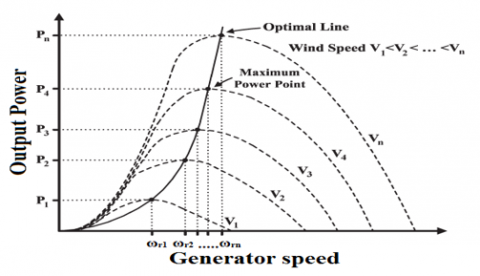Figure 5. WT output power versus wind speeds with MPPT curve

## 6.pngFigure 6. The turbine power versus rotor speed from 3 to 26 m/s

The MPPT curve is calculated from the WT power Eq. 2 after modifying it with the optimal values for λopt=8.1 and βopt = 0.0, and then given by:

$\mathrm{P}_{\mathrm{opt}}=\frac{1}{2} \rho \mathrm{AC}_{\mathrm{p}}^{\mathrm{opt}}\left(\lambda_{\mathrm{opt}}\right) \mathrm{v}_{\mathrm{w}}^{3}=\frac{1}{2} \rho \mathrm{AC}_{\mathrm{p}}^{\mathrm{opt}}\left(\lambda_{\mathrm{opt}}\right)\left(\frac{\omega_{\mathrm{t}} \mathrm{R}}{\lambda_{\mathrm{opt}}}\right)^{3}$  (5)

Thus, the target power Popt is proportional to the $\mathrm{V}_{\mathrm{W}}^{3}$ or to the cube of the rotor speed as written in Eq. 5. The proposed strategy is to adjust the generator speed to produce maximum power while the wind speed varied. The rotor should be operated at ωrn (reference rotor speed) which is derived from effective wind speed using Eq. 1 of the TSR. TSR value is constant (equal to 8.1 for the WT under study) for all MPPs, while the generator rotational speed is related to the wind speed by:

$\omega_{\mathrm{t}-\mathrm{ref}}=\frac{\lambda_{\mathrm{opt}} \mathrm{v}_{\mathrm{n}}}{\mathrm{R}}$      (6)

$\omega_{\mathrm{rn-ref}}=\omega_{\mathrm{t}-\mathrm{ref}} n_{\mathrm{gear}}=\frac{\lambda_{\mathrm{opt}} \mathrm{n}_{\mathrm{gear}}}{\mathrm{R}} \mathrm{V}_{\mathrm{n}}$ (7)

The achieved VS range at the WT shaft or generator axes differs from the fixed frequency of the power system which 50Hz or 60Hz. Direct coupling of a synchronous generator to the grid is therefore not possible, and the induction machine is too small in speed variation possibilities (<1 %). Thus, the presence of a power converter is very important for VSWT. A schematic diagram for the control system based on WS that measured by the anemometer shown in Figure 7.

## 7.png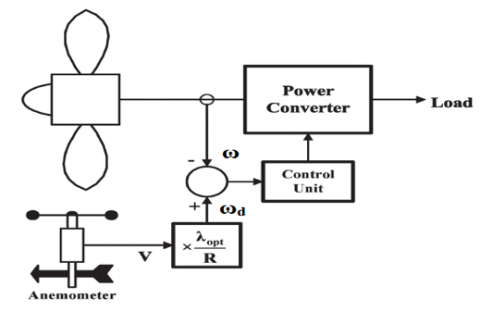Figure 7. MPPT method control system based on rotating-speed measurements

The reference generator speed corresponding to the optimal value of specific speed λopt =8.1 are calculated from Eq. 7. The rotor speed is controlled by the speed of the controller that regulates the generator electrical power, and hence the generator torque, according to the optimal specified speed λopt by imposing on the generator torque to equal to its reference value. Controller speed can be applied by using a PI controller which contains two variable gains, the first gain is integral gain KI while the second gain is proportional gain KP. The speed error is used as an input to the PI controller. Then the output of the PI controller is the reference generator torque (Te-ref) which maximizes the power. The gains of the PI controller is determined using a trial and error method.

$\mathrm{T}_{\mathrm{e}-\mathrm{ref}}=\mathrm{k}_{\mathrm{p}} \mathrm{e}+\mathrm{k}_{\mathrm{i}} \int \mathrm{e} \mathrm{dt}$   (8)

$\mathrm{e}=\omega_{\mathrm{r}-\mathrm{ref}}-\omega_{\mathrm{r}}$            (9)

Then this reference electromagnetic torque is applied to the generator. The dynamic Equation of WT with the generator in Eq. 10 can be rearranged as in Eq. 11.

$\frac{\mathrm{d} \omega_{\mathrm{r}}}{\mathrm{dt}}=\frac{\mathrm{P}}{2}\left(\frac{\mathrm{d} \omega_{\mathrm{m}}}{\mathrm{dt}}\right)=\frac{\mathrm{P}}{2 \mathrm{I}}\left(\mathrm{T}_{\mathrm{mech}}-\mathrm{T}_{\mathrm{em}}\right)-\frac{\beta}{\mathrm{I}} \omega_{\mathrm{r}}$   (10)

$\mathrm{T}_{\mathrm{t}}-\mathrm{T}_{\mathrm{e}-\mathrm{ref}}=\frac{2}{\mathrm{P}}\left(J \frac{\mathrm{d} \omega_{\mathrm{r}}}{\mathrm{dt}}+\mathrm{D} \omega_{\mathrm{r}}\right)$   (11)

And then,

$\omega_{\mathrm{r}}=\frac{\frac{\mathrm{P}}{2}\left(\mathrm{T}_{\mathrm{t}}-\mathrm{T}_{\mathrm{e}-\mathrm{ref}}\right)}{\mathrm{Js}+\mathrm{D}}$           (12)

The strategy of MPPT control is described in the block diagram that describes, which achieved by using generator torque control as shown in Figure 8. In this diagram, the Equations of the PI controller (Eqns 8 and 9) are combined with the dynamic equation of the generator (12) to obtain the generator torque command.

## 8.pngFigure 8. The proposed control strategy block diagram in region 2

5. Above Rated Wind Speed (Region 3) Control Strategy

On contrary to region 2 which requires maximizing the energy captured from the wind by maximizing CP, a VSWT in region 3 often maintains a constant speed and constantly rated power by decreasing CP to avoid the excess power that exists in high wind speed. This can be achieved by yaw control, GTC, and BPC strategies. All these control strategies used to limit the WT’s energy capture.

Efficient and reliable operation of a WECS heavily relies on the control systems applied on the WT operating at different regions. Pitch angle control is applied for region 3 to limit the power captured from the wind by the WT. Any changes in pitch angle can affect the output power. The main advantage of such a system is the controlling of aerodynamic loads and hence decreasing the load on the WT drivetrain. Since loads are decreased, the whole drivetrain can be designed accordingly and the cost for the drivetrain and mechanical suspension devices are, thus, also decreased. The blade pitch is a simple and effective method for limiting speed and power. In some machines, independent blade pitch control is adjusted independently of the other blades; in others, the pitch angle of each blade is adjusted identically (rotor collective pitch control).

Figure 3 shows that if the value of β increases, the value of CP decreases. Thus, the VSWT is usually equipped with pitch control, where the blade can be turned to increase or decrease lift forces on the blade profile and thereby continuously controls energy absorption from the wind.   Then, by adjusting the pitch angle of the blades it becomes the effective means of limiting WT performance at strong wind speeds. So we must be awarded the pitch system.

A pitch system monitors and adjusts the angle of the WT blades and thus controls the rotation of the rotor speed. The pitch angle control used to accelerate the rotor rotation speed at lower wind speed while reduces the rotor rotation speed at higher wind speed. Over a certain wind speed (cut-out speed) the pitch system starts to rotate the blades out of the wind, thereby slowing and stopping the rotor to avoid damage. To put the blades into the desired position an Electric or hydraulic pitch servos are employed.

Numerous control methods have been applied to design pitch angle controllers, such as PI-type controller. Typically, a WT pitch control is built with a controller, pitch control mechanisms and feedback module monitoring output power. The controller used in the present study is a classical technique PI controller. The PI is then used to control the pitch angle of the blade to limit the electric power output and speed on both the low-speed shaft and high-speed shaft to their rated values for WTs operated at region 3. Figure 9 illustrate the control system block diagram of the PI controller contains two gains KP and KI. The generator speed error is used as an input for the PI controller. Then the PI controller output is used to actuate the blade pitch angle (β) which is maintained the generator speed and frequency at their rated value. The PI controller gains are determined by trial and error method. The PI controller expressed as follows:

$\beta_{\mathrm{d}}=\mathrm{k}_{\mathrm{p}} \mathrm{e}+\mathrm{k}_{\mathrm{i}} \int \mathrm{e} \mathrm{dt}$ (13)

$\mathrm{e}=\omega_{\mathrm{r}-\mathrm{ref}}-\omega_{\mathrm{r}}$ (14)

## 9.pngFigure 9. Block diagram for the proposed control strategy at region 3

However, the WT is a highly non-linear system due to its nonlinear aerodynamics. As the WT contains strong aerodynamic non-linear and operates under wind speed time-varying, the linear PI with fixed gains based on one operation point cannot provide satisfactory performance in the whole wind speed region where operation points shift from that normal point. Advanced control methods have been proposed to tackle this problem, like as the gain scheduling PI (GS-PI) .

In GS-PI strategy the PI gains of the controller are always modified to track the new operating points. Figure 10 shows the control block diagram of the PI and GS-PI controller. The scheduled gain pairs are obtained by multiplying the constant PI gains with a scheduled gain Kβ that is a function of pitch angle (β) as given in Eq. 16. Kβ value is set to be 1 in the case of the conventional PI controller. To obtain a continuous pitch angle based switching, the scheduled gain Kβ is proposed to compensating the variation of the aerodynamic sensitivity and is given as:

$\mathrm{K}_{\beta}=\left\{\begin{array}{lr}{-0.001 \beta^{2}+0.01 \beta+1} & {0^{\circ}<\beta \leq 30^{\circ}} \\ {1} & {\beta>30}\end{array}\right.$ (15)

$\beta_{\mathrm{d}}=\mathrm{K}_{\beta}\left(\mathrm{k}_{\mathrm{p}} \mathrm{e}+\mathrm{k}_{\mathrm{i}} \int \mathrm{e} \mathrm{d} \mathrm{t}\right)$ (16)

## 10.pngFigure 10. Block diagram of GS-PI controller

6. Simulation Results for the VSWT

## 11.pngFigure 11. WECS with pitch control Simulink model

This section concentrated on the controllers applied for regions 2 and 3. The limits for these regions determined by the values of cut-in, rated and cut-out of wind speeds. The choice of cut-in, rated and shutdown wind speeds are made by the WT designer who will try to balance maximum energy extraction with controlling the mechanical loads and hence the capital cost of the WT. In this paperwork, the WT is started at WS of 4 m/s and stopped at 25 m/s. The designed rated WS is 12 m/s. Therefore, region 2 starts from 4 m/s wind speed up to12 m/s, while region 3 from 12 m/s up to 25 m/s.

Figure 11 shows the overall Matlab Simulink model of the system under study including torque and blade pitch control. The turbulent wind disturbances by the realistic wind model and also random wind speed profile variation model are used for control performance evaluation proposes.

6.1 Simulation results for region 2

At first, the proposed WECS is studied for wind speed varies under region 2 which in our case study varies from 4 to 12 m/s. The proposed control strategy for this region is generator torque control which achieves the required MPPT objective. Figure 12 shows the Simulink model of the generator torque control. MPPT strategy is required through region 2, and hence it is simulated for wind speeds available in this region from 4 to 12 m/s. Figure 13 shows the power curves for the proposed WT with the MPPT curve. The MPPT curve is simulated by applying Eq. 5 to obtain the maximum available power in the wind at each wind speed.

## 12.pngFigure 12. The generator torque control Simulink model

## 13.pngFigure 13. The WT power curves with MPPT curve

The response of the system under this range of variable wind speeds without any control strategy applied is studied through the TSR and power coefficient (Cp) performance as seen in Figures 14 and 15. It is observed that the TSR is high at lower wind speeds and the power coefficient is low, so the system can't capture the available maximum power from the wind.

The objective in this region of the controller is to maximize the captured power, and hence maintaining Cp constant at its maximum value 0.48. In other words, the TSR must be maintained at its optimal value of 8.1. In addition, the operating generator speed must track the reference rotation speed represented in Figure 13 to achieve maximum power tracking objective. Therefore, the response of the proposed WECS through this region will usually be studied usually by studying the performance of Cp, TSR, and generator operation rotational speed with respect to the reference rotational speed under wind speed variation.

## 14.pngFigure 14. The TSR curve through region 2 without any control strategy

## 15.pngFigure 15. Region 2 power coefficient without any control strategy

## 16.pngFigure 16. Realistic wind speed profile that used to study the response of the system

The proposed controller has to be implemented, tested and if necessary modified so that the output response desired of the WT parameters are achieved during the simulation. The controller needs to be tuned according to the specific system that it is getting used for, which is in our case 6.3 MW WT. PI controller parameters are implemented by using KP = 10 and KI = 20. Two wind speed profiles of variation will be used in studying the performance of the strategy through region 2 in order to prove its robustness for any wind speed variation. Figure 5.16. Shows the first realistic wind speed profile variation while the other wind speed profile changes randomly during the range of region 2 as shown in Figure 17. WECS response is also studied under balanced and unbalanced excitation process for the SEIG.

## 17.png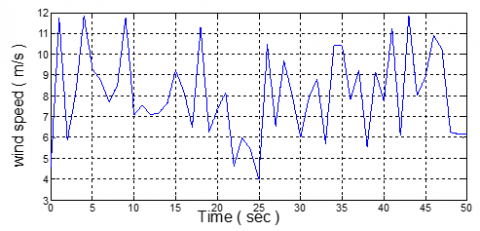Figure 17. Random wind speed profile variation between 4 and 12 m/s

6.1.1 Results of the system used realistic wind speed profile

The first case study is to study the performance of the system with RL-load (R =100 Ω and L=0.12H) under balanced excitation for the SEIG. $\mathrm{C}_{\mathrm{P}}$ and $\lambda$ curves when the real wind profile of Figure 16 is applied shown in Figures 18 and 19, respectively. It is observed from the curves that Cp remains constant and equal to its maximum value of 0.48. TSR is also approximately constant around its optimal value which is 8.1.

## 18.pngFigure 18. Cp curve for RL-load with balanced excitation

## 19.pngFigure 19. The TSR curve for RL-load with balanced excitation

Figure 20 shows the reference generator rotational speed and the operating rotational speed (in rad/s). This reference rotational speed is the high-speed shaft at which the maximum power can be obtained as shown for MPPT curve in Figure 13. The generator speed that achieved by the controller is identical to the required reference speed as shown in Figure 20 and prove the proposed controller effectiveness.

## 20.pngFigure 20. The operating generator rotational speed with the required reference speed

## 21.pngFigure 21. The applied wind speed to study the performance of the WECS in region2

## 22.pngFigure 22. Cp performance due to step variation of wind speed

## 23.pngFigure 23. TSR performance due to step variation of wind speed

Figure 16 shows the applied real wind speed variation and the generator operating speed corresponding to every wind speed can't be observed clearly. Therefore, to distinguish between the operating rotational speed corresponding to each wind speed during region 2 from 4 to 12 m/s, the proposed response will be studied by applying a step variation to the wind speed by about 2 seconds as shown in Figure 21.

Figures 22 and 23 show the Cp and $\lambda$ performances due to the wind speed of Figure 21. It is observed that the Cp value remains constant at its maximum value and TSR is also constant at its optimal value of 8.1. In case of step wind speed variation, there are impulses at each wind speed that represent transients due to the sudden change.

A comparison between the operating generator speed and the reference rotational speed at the high-speed shaft (in rpm) is introduced in Figure 24. It is observed that the operating speed is identical to the required reference speed. That means the generator operates at the rotation speed which achieves maximum power for each wind speed. It is observed also that there is a unique rotation speed that can achieve the maximum power captured from the wind for each wind speed. Figure 13 compares the rotational speed with the desired speed. In addition to the voltage, the response is introduced in Figure 25. It is observed that the voltage is not constant since the generator rotational speed is not constant also but varies with wind speed. Then, VSWT requires power converter to regulate the voltage and can be coupled with the grid. The resultant voltage is the appropriate voltage for each speed.

## 24.pngFigure 24. Operating generator and reference rotation speeds for step wind variation

## 25.png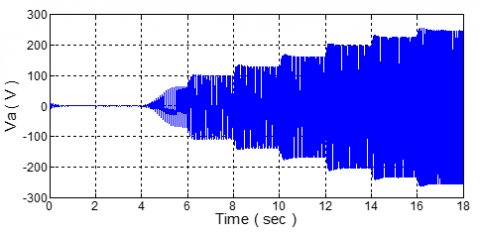Figure 25. The output phase voltage due to step wind speed variation

## 26.pngFigure 26. Comparison between WT power with and without a controller

Figure 26 shows the output power of the WT with and without the presence of generator torque control compared. The response is also studied under unbalanced excitation for the SEIG. Performance of the system response is introduced through Figures 27-29.

## 27.png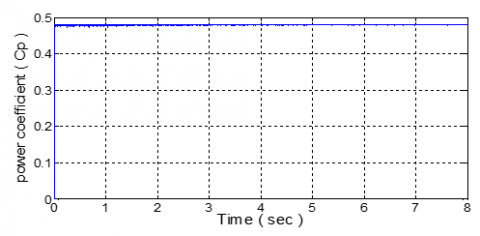Figure 27. The Cp performance under unbalanced excitation

## 28.pngFigure 28. TSR performance under unbalanced excitation

## 29.pngFigure 29. Generator speed with reference speed under unbalanced excitation

6.1.2 Results of the system used random variation wind speed profile

Another strategy for wind speed variation is applied for the system which is introduced in Figure 17. This wind speed varies randomly from 4 to 12 m/s. The system performance is introduced through Figures 30-32.

## 30.pngFigure 30. Power coefficient performance under random variation for wind speed

## 31.pngFigure 31. TSR performance under random variation for wind speed

## 32.pngFigure 32. The operating generator speed with the required reference speed

The response of the system is also studied with unbalanced excitation for the SEIG. The system performance is introduced through Figures 33 and 34.

## 33.pngFigure 33. Cp performance under random variation and unbalanced excitation

## 34.pngFigure 34. Generator speed under random variation and unbalanced excitation

6.2 Region 3 simulation results

Secondly, the proposed WECS is studied for wind speed varies above the rated speed (region 3), so wind speed varies from 12 up to 25 m/s the cut-out speed. The proposed control strategy for this region is pitch angle control to limit the power captured by the WT to its rated value which in our case study is 6.4 kW. First, the conventional PI controller is applied for the proposed WECS without real speed model to study the effects of the controller and decide whether the modification introduced in Eqns. 15 & 16 is needed or not. Figure 35 shows the Simulink model of the conventional PI-pitch angle controller and the applied wind speed profile without real speed model shown in Figure 36. Wind speed starts from 11 m/s as an initial condition to simulate the realist and ends at 25 m/s.

## 35.png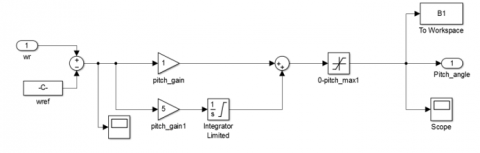Figure 35. Pitch angle control Simulink model using a PI controller

## 36.pngFigure 36. Mean wind speed varies from 11 up to 25 m/s

WECS response due to the proposed wind speed in Figure 36 is shown through Figures 37-42.

## 37.png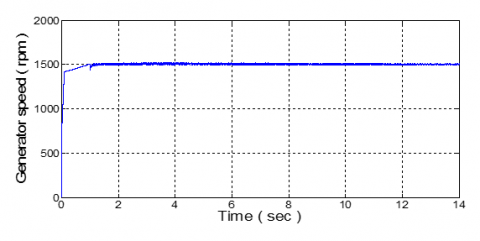Figure 37. The generator rotational speed under mean speed varies from 11-25 m/s

## 38.pngFigure 38. The frequency of the system under mean wind speed varies from 11-25 m/s

## 39.pngFigure 39. The generated voltage under mean wind speed varies from 11-25 m/s

## 40.pngFigure 40. The pitch angle of the blade under mean wind speed varies from 11-25 m/s

## 41.pngFigure 41. Power coefficient performance under mean speed varies from11-25 m/s

## 42.png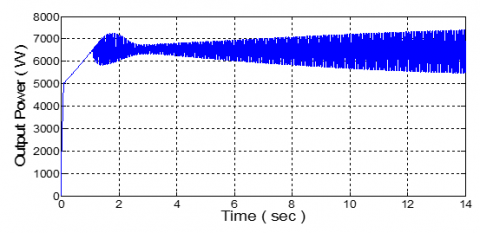Figure 42. The WT output power under mean wind speed varies from 11-25 m/s

## 43.pngFigure 43. The $\beta$ angle after applying GS-PI controller

## 44.pngFigure 44. The power coefficient performance after applying GS-PI controller

## 45.pngFigure 45. The WT output power after applying GS-PI controller

It is observed that the generator rotational, the frequency and the voltage are stable and constant at their rated value despite the wide range of variable wind speed used. However, pitch angle, power coefficient, and output power have strong oscillations that increased as the wind speed increases. So the controller system had to be modified to be more efficient for all wind speeds at this region by applying GS-PI controller, which modifies the controller gains as the wind speed variation. Figures 43-45 show the response of the system after applying the modified PI- controller.

It is observed that power output is more stable at its rated value and have small oscillations around its rated value. In addition, the β angle hasn't any oscillation and increases as wind speed increases. The β angle is also constant at zero value during speeds 11 and 12 m/s. However, it starts to take values after speeding of 12 m/s. The power coefficient is constant at its maximum value which is 0.48 during speeds 11 and 12 m/s to capture maximum power and then starts to decreased to limit the power captured at its rated value. The output power starts to increase the rated power which may cause failure to the system.

After adjusting the controller for mean wind speed, the proposed controller is applied for the original system with real wind speed profile. The applied wind speed is shown in Figure 46. The response of the system under this wind speed for conventional PI- controller and GS-PI controller is introduced through Figures 47-55.

## 46.pngFigure 46. The real wind speed profile from 11to 25 m/s

## 47.pngFigure 47. The generator rotational speed under real wind speed with conventional PI

## 48.pngFigure 48. The frequency of the system under real wind speed with conventional PI

## 49.pngFigure 49. The generated voltage under real wind speed with conventional PI

## 50.pngFigure 50. The pitch angle of blades under real wind speed with conventional PI

## 51.pngFigure 51. Power coefficient performance under real wind speed with conventional PI

## 52.pngFigure 52. The WT output power under real wind speed with conventional PI

## 53.pngFigure 53. The pitch angle under real speed with applying GS- PI

## 54.pngFigure 54. Power coefficient performance under real speed with applying GS- PI

## 55.pngFigure 55. The WT output power under real wind speed with applying GS- PI

The comparison between rated power, conventional PI- controller, and gain scheduled PI-controller output power of the WT is shown in Figure 56.

## 56.pngFigure 56. Comparison between rated power, PI, and GS-PI output power

## 57.png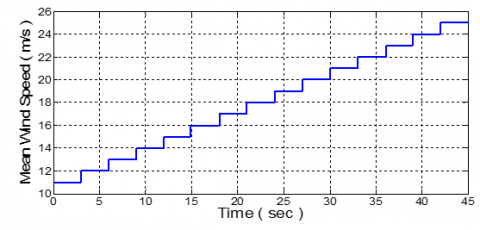Figure 57. Mean Wind speed varies as a step from 11 up to 25 m/s

## 58.pngFigure 58. Pitch angle for wind speeds vary as a step from 11 up to 25 m/s

## 59.pngFigure 59. Power coefficient for wind speeds vary as a step from 11 to 25 m/s

## 60.pngFigure 60. The WT output power for wind speeds vary as a step from 11 to 25 m/s

In order to discriminate the pitch angle value for each wind speed and represent the power coefficient performance under different wind speeds, Figure 57 shows a step with about 3 seconds of each speed. The pitch angle, power coefficient performance, and output power are introduced in Figures 58, 59 and 60, respectively.

Figure 61 shows the overall power curve with respect to wind speed for typical WT of Figure 2 for all regions of the proposed WECS studied in this paperwork.

## 61.png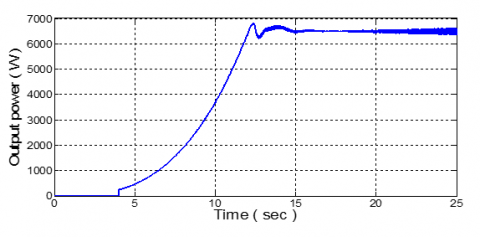Figure 61. The output power curve with respect to wind speed from 0 to 25 m/s

7. Conclusion

VSWT is more favorable than FSWT due to its efficient to capture more wind power and achieve higher power qualities. VSWT have three main regions of operations. The control is very important for VSWT to maximize the picked up power from the wind in region 2 and Limit the captured power in region 3. The proposed control strategy for region 2 is generator torque control to make MPPT. When the power control scheme is applied to the proposed WECS both generator and turbine torques are adapted to the load power when the wind varies. Simulation results have shown good performances of the proposed control system. The proposed control strategy for region 3 is the pitch angle control. A conventional PI-controller is first applied to the proposed WECS in order to study and evaluate its effectiveness for the WT under study. Since the WT is a highly non-linear system due to its nonlinear aerodynamics, the conventional PI-controller achieves the objective but with some disturbances. Therefore, the PI-controller is modified by a strategy known as gain scheduled in which the controller gains are modified continuously as the wind speed varies. The proposed GS-PI controller achieves a satisfactory performance as shown from the simulation results. A comparison between the system performance used traditional PI-controller and gain scheduled PI- controller is done. Simulation results prove the robustness of the system and the possibility of obtaining constant voltage, constant rotational speed and limit the output power at its rated value for all wind speeds in region 3. The power curve for the proposed WT is drawn for all regions (wind speed varies from 4 up to 25 m/s).

References

 Anaya-Lara, O., Jenkins, N., Ekanayake, J., Cartwright, P., Hughes, M. (2009). Wind energy generation: modelling and control.

 Jonkman, J.M., Buhl, J.M.L. (2005). FAST User’s Guide, National Renewable Energy Laboratory.

 Bianchi, F.D., Battista, H.D., Ricardo J. Mantz (2007). Wind Turbine Control Systems: Principles, Modelling and Gain Scheduling Design. Springer-Verlag. https://doi.org/WO2011115843 A1

 Yang, W., Yang, J. (2019). Advantage of variable-speed pumped storage plants for mitigating wind power variations: Integrated modelling and performance assessment. Applied Energy, 237: 720-732. https://doi.org/10.1016/j.apenergy.2018.12.090

 Yin, M., Yang, Z., Xu, Y., Liu, J., Zhou, L. (2018). Aerodynamic optimization for variable-speed wind turbines based on wind energy capture efficiency. Applied Energy, 221: 508-521. https://doi.org/10.1016/j.apenergy.2018.03.078

 Gambier, A., Nazaruddin, Y.Y. (2018). Collective Pitch Control with Active Tower Damping of a Wind Turbine by Using a Nonlinear PID Approach. IFAC-PapersOnline, 51(4): 238-243. https://doi.org/10.1016/j.ifacol.2018.06.072

 Golnary, F., Moradi, H. (2018). Design and comparison of quasi continuous sliding mode control with feedback linearization for a large-scale wind turbine with wind speed estimation. Renewable Energy, 127: 495-508. https://doi.org/10.1016/j.renene.2018.04.081

 Leisten, C., Jassmann, U., Balshüsemann, J., Abel, D. (2018). Model Predictive Speed Control of a Wind Turbine System Test Bench. IFAC PapersOnLine, 51: 349-354. https://doi.org/10.1016/j.ifacol.2018.11.408

 Abdullah, M.A., Al-Hadhrami, T., Tan, C.W., Yatim, A.H. (2018). Towards Green Energy for Smart Cities: Particle Swarm Optimization Based MPPT Approach. IEEE ACCESS, 6: 58427-58438. https://doi.org/10.1109/ACCESS.2018.2874525

 Lin, Z., Chen, Z., Liu, J., Wu, Q. (2019). Coordinated mechanical loads and power optimization of wind energy conversion systems with variable-weight model predictive control strategy. Applied Energy, 236: 307-317. https://doi.org/10.1016/j.apenergy.2018.11.089

 Rezaeiha, A., Kalkman, I., Blocken, B. (2017). Effect of pitch angle on power performance and aerodynamics of a vertical axis wind turbine. Applied Energy, 197: 132-150. https://doi.org/10.1016/j.apenergy.2017.03.128

 Yin, M., Yang, Z., Xu, Y., Liu, J., Zhou, L., Zou, Y. (2018). Aerodynamic optimization for variable-speed wind turbines based on wind energy capture efficiency. Applied Energy, 221: 508-521. https://doi.org/10.1016/j.apenergy.2018.03.078

 Fathabadi, H. (2016). Novel high-efficient unified maximum power point tracking controller for hybrid fuel cell/wind systems. Applied Energy, 183: 1498-1510. https://doi.org/10.1016/j.apenergy.2016.09.114

 Song, D., Yang, J., Cai, Z., Dong, M., Su, M., Wang, Y. (2017). Wind estimation with a non-standard extended Kalman filter and its application on maximum power extraction for variable speed wind turbines. Applied Energy, 190: 670-685. https://doi.org/10.1016/j.apenergy.2016.12.132

 Bizon, N. (2018). Optimal operation of fuel cell/wind turbine hybrid power system under turbulent wind and variable load. Applied Energy, 212: 196-209. https://doi.org/10.1016/j.apenergy.2017.12.034

 Saleh, S.M.S. (2016). Study of wind turbine based self-excited induction generator under nonlinear resistive loads as a step to solve the Egypt electricity crisis. Computers and Electrical Engineering, 51: 1-11. https://doi.org/10.1016/j.compeleceng.2016.02.018

 Farag, A.S., Saleh, S.M., El-Bayoumi, G. (2017). Pitch Control dynamic study of isolated wind turbine based self-excited induction generator under realistic wind speed profiles. 2017 Nineteenth International Middle East Power Systems Conference (MEPCON). https://doi.org/10.1109/MEPCON.2017.8301212

 Lee, K.Y., Tsao, S.H., Tzeng, C.W., Lin, H.J. (2018). Influence of the vertical wind and wind direction on the power output of a small vertical-axis wind turbine installed on the rooftop of a building. Applied Energy, 209: 383-391. https://doi.org/10.1016/j.apenergy.2017.08.185

 Melício, R., Mendes, V.M.F., Catalão, J.P.S. (2011). Transient analysis of variable-speed wind turbines at wind speed disturbances and a pitch control malfunction. Applied Energy, 88(4): 1322-1330. https://doi.org/10.1016/j.apenergy.2010.10.021

 Parwal, A., Fregelius, M., Temiz, I., Göteman, M., de Oliveira, J.G., Boström, C., Leijon, M. (2018). Energy management for a grid-connected wave energy park through a hybrid energy storage system. Applied Energy, 231: 399-411. https://doi.org/10.1016/j.apenergy.2018.09.146

 Song, D., Fan, X., Yang, J., Liu, A., Chen, S., Joo, Y.H. (2018). Power extraction efficiency optimization of horizontal-axis wind turbines through optimizing control parameters of yaw control systems using an intelligent method. Applied Energy, 224: 267-279. https://doi.org/10.1016/j.apenergy.2018.04.114

 Khalid, M., Aguilera, R.P., Savkin, A.V., Agelidis, V.G. (2018). On maximizing profit of wind-battery supported power station based on wind power and energy price forecasting. Applied Energy, 211: 764-773. https://doi.org/10.1016/j.apenergy.2017.11.061

 Kumar, D., Chatterjee, K. (2016). A review of conventional and advanced MPPT algorithms for wind energy systems. Renewable and Sustainable Energy Reviews, 55: 957-970. https://doi.org/10.1016/j.rser.2015.11.013

 Gao, J. (2004). Robust Control Design of Gain-scheduled Controllers for Nonlinear Processes.